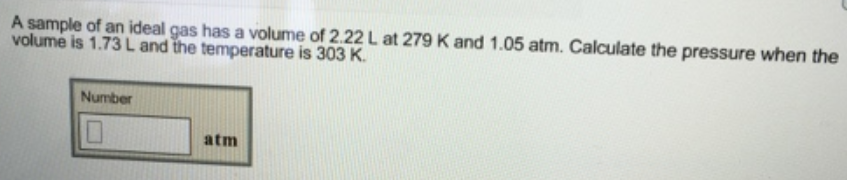Chemistry Practice Problems Chemistry Gas Laws Practice Problems Solution:  A sample of an ideal gas has a volume of 2.22 L a...

# Solution:  A sample of an ideal gas has a volume of 2.22 L at 279 K and 1.05 atm. Calculate the pressure when the volume is 1.73 L and the temperature is 303 K.

###### Problem

A sample of an ideal gas has a volume of 2.22 L at 279 K and 1.05 atm. Calculate the pressure when the volume is 1.73 L and the temperature is 303 K.View Complete Written Solution

Chemistry Gas Laws

Chemistry Gas Laws

#### Q. An ideal gas is allowed to expand from 7.60 L to 72.2 L at constant temperature. By what factor does the volume increase? The pressure will increase b...

Solved • Tue May 08 2018 09:05:34 GMT-0400 (EDT)

Chemistry Gas Laws

#### Q. What is the volume occupied by 18.6 g of argon gas at a pressure of 1.19 atm and a temperature of 394 K? Express your answer with the appropriate unit...

Solved • Tue Apr 24 2018 10:49:07 GMT-0400 (EDT)

Chemistry Gas Laws

#### Q. A 12 liter tank contains helium gas pressurized to 160 atm. What size tank would be needed to contain the same amount of helium at atmospheric pressur...

Solved • Fri Mar 30 2018 15:36:16 GMT-0400 (EDT)

Chemistry Gas Laws

#### Q. A 12 Liter tank contains helium gas pressurized to 160 atm.How many 3-liter balloons could the 12 Liter helium tank fill?(Keep in mind that an "exhaus...

Solved • Tue Mar 27 2018 11:49:05 GMT-0400 (EDT)